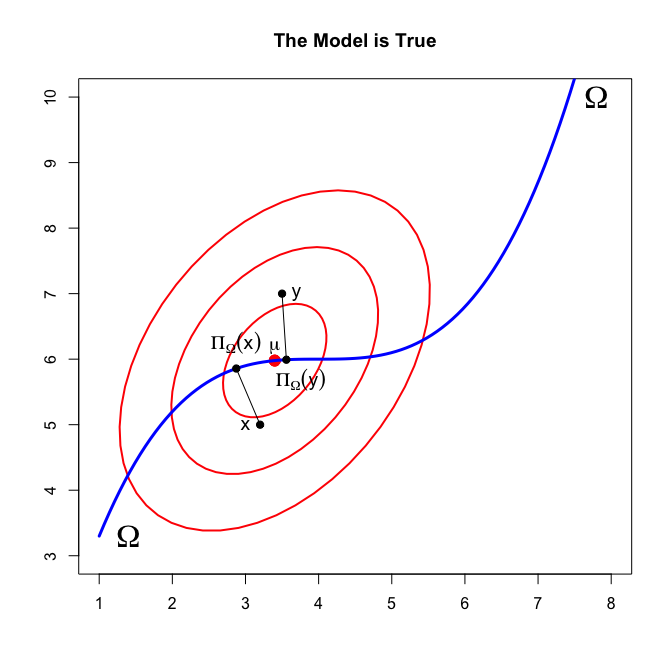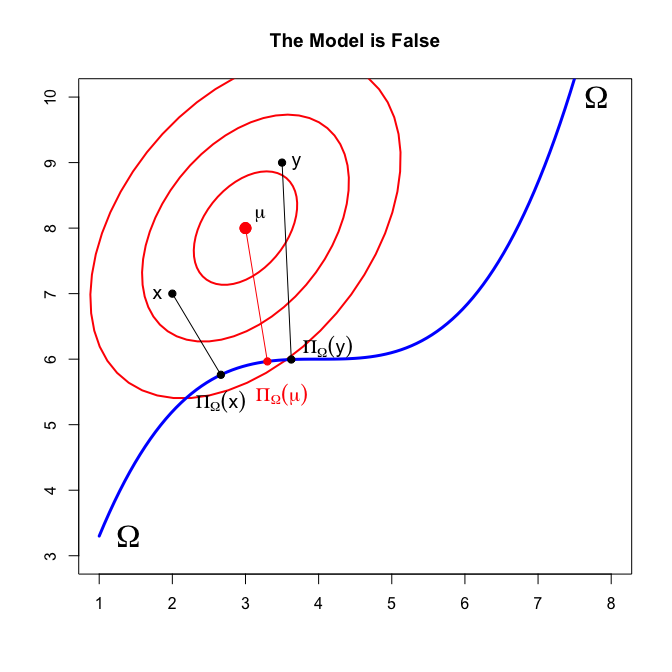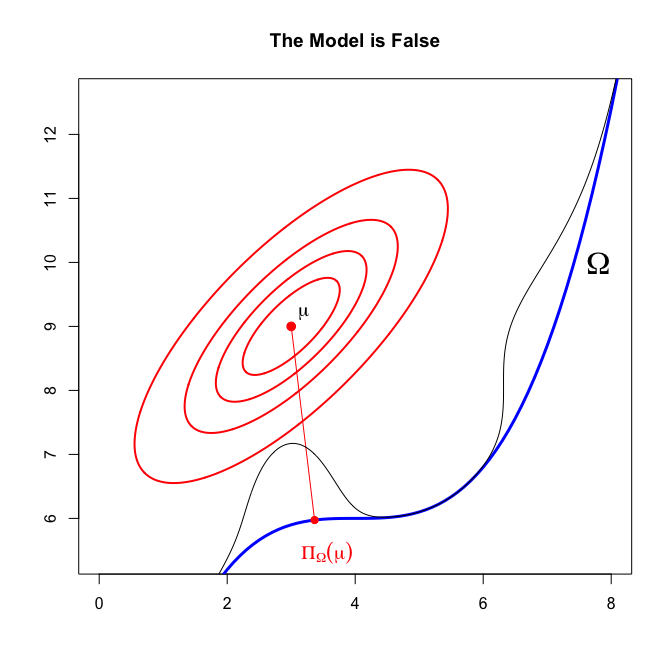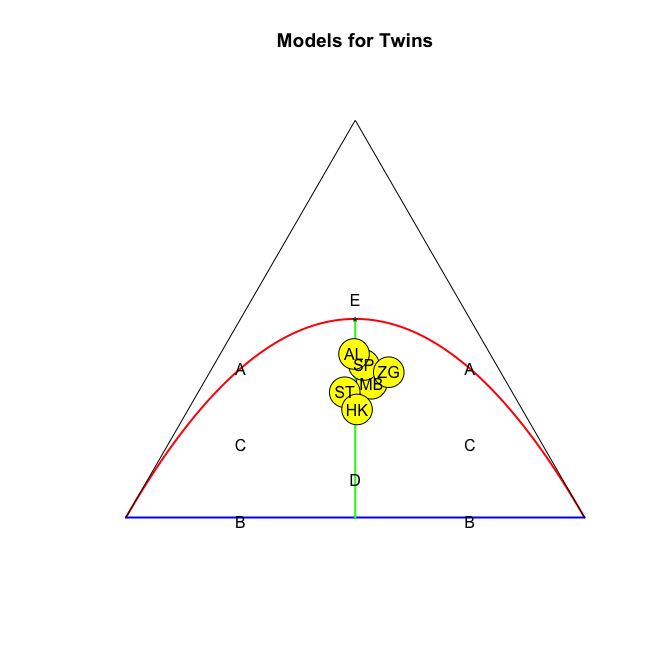$\newcommand{\defi}{\mathop{=}\limits^{\Delta}} % mathop for define \def\Rav#1{\underline #1} % random variables are underlined \def\Exp#1{{\bf E}\,(#1)} % expected values are bold \def\Var#1{{\bf V}\,(#1)} % variances are bold \def\Cov#1#2{{\bf C}\,(#1,#2)} % covariances are bold \def\Cor#1#2{{\bf R}\,(#1,#2)} % correlations are bold \def\Rx{\Rav{x}} % random variables are underlined \def\Ry{\Rav{y}} \def\Rz{\Rav{z}} \def\Rp{\Rav{p}} % and so are random proportions \def\Rq{\Rav{q}} \newcommand{\mR}{\mathbb{R}} % reals \newcommand{\mN}{\mathbb{N}} % natural numbers \newcommand{\mS}{\mathbb{S}} % unit simplex \newcommand{\mC}{\mathbb{C}} % complex numbers \newcommand{\mQ}{\mathbb{Q}} % rational numbers \newcommand{\mZ}{\mathbb{Z}} % integers \newcommand{\cA}{\mathcal{A}} \newcommand{\cB}{\mathcal{B}} \newcommand{\cC}{\mathcal{C}} \newcommand{\cD}{\mathcal{D}} \newcommand{\cE}{\mathcal{E}} \newcommand{\cF}{\mathcal{F}} \newcommand{\cG}{\mathcal{G}} \newcommand{\cH}{\mathcal{H}} \newcommand{\cI}{\mathcal{I}} \newcommand{\cJ}{\mathcal{J}} \newcommand{\cK}{\mathcal{K}} \newcommand{\cL}{\mathcal{L}} \newcommand{\cM}{\mathcal{M}} \newcommand{\cN}{\mathcal{N}} \newcommand{\cO}{\mathcal{O}} \newcommand{\cP}{\mathcal{P}} \newcommand{\cQ}{\mathcal{Q}} \newcommand{\cR}{\mathcal{R}} \newcommand{\cS}{\mathcal{S}} \newcommand{\cT}{\mathcal{T}} \newcommand{\cU}{\mathcal{U}} \newcommand{\cV}{\mathcal{V}} \newcommand{\cW}{\mathcal{W}} \newcommand{\cX}{\mathcal{X}} \newcommand{\cY}{\mathcal{Y}} \newcommand{\cZ}{\mathcal{Z}} \newcommand{\del}{\delta} \newcommand{\gam}{\gamma} \newcommand{\lbd}{\lambda} \newcommand{\ome}{\omega} \newcommand{\eps}{\epsilon} \newcommand{\sig}{\sigma} \newcommand{\alp}{\alpha} \newcommand{\bet}{\beta} \newcommand{\Del}{\Delta} \newcommand{\Gam}{\Gamma} \newcommand{\Lbd}{\Lambda} \newcommand{\Ome}{\Omega} \newcommand{\Eps}{\Epsilon} \newcommand{\Sig}{\Sigma} \newcommand{\Alp}{\Alpha} \newcommand{\vat}{\vartheta} \newcommand{\bul}{\bullet} \def\claw{\mathop{\Longrightarrow}\limits^{\cL}} \def\cprob{\mathop{\Longrightarrow}\limits^{\cP}} \def\isad{\mathop{=}\limits^{a.d.}}$Note: This is a working paper which will be expanded/updated frequently. The directory gifi.stat.ucla.edu/truth has a pdf copy of this article, the complete Rmd file with all code chunks, and R and C files with the code.

# 1 Introduction

In this paper, I distinguish various types of errors an estimate can have, and I estimate these errors using Delta method and Jackknife techniques. The paper is based on, but extends and generalizes, De Leeuw (1988) and De Leeuw (1997).

The notion of a distance-type loss function plays a central role. I evaluate the distance between the target and the sequence of estimates. This is called the overall error. I also compute the distance between the estimates and a sequence of variables with the same distribution as the target sequence, but independent of the target sequence. This is called the prediction error. Then I compute estimates of the distance between the target and the limit of the estimation sequence, which is the specification error. Finally, in the computations I need the distance between the target sequence and the estimation sequence, which I call the deviance. Just words, of course, but chosen to suggest the various types of errors that are involved.

The paper is closely related to the work of Linhart and Zucchini (1986), which has been used by Cudeck and Browne (1983) and Browne and Cudeck (1989), Browne and Cudeck (1992). A related Bayesian approach, also based on predictive distribution, has been discussed by Gelfand, Dey, and Chang (1992). Related research in covariance structure modeling has been published by McDonald (1989), McDonald and Marsh (1990), and Bentler (1990).

# 2 Estimation Problem

We start, as in De Leeuw , with simple experiments in which we estimate a vector of proportions (this could also be a multidimensional cross table, or a discreticized multivariate distribution). This will be generalized slightly in a later section.% Thus we start by considering an with the following ingredients. % Definition: The is sequence of random vectors of $$\underline{p}_n,$$ taking values in the unit simplex $$\mathbb{S}^m.$$ The is a fixed element $$\pi$$ of $$\mathbb{S}^m.$$

Of course this is highly suggestive, and to some extent misleading, terminology. Actual data are not a random variable, and certainly not a sequence of random variables. To arrive at this representation we must first embed the actual data in a , which is modeled by a single random variable, and we then have to embed that random variable in a sequence to get our asymptotics under way. Thus the distance from what we call to what an ordinary person would call is quite large. In the same way calling a particular point in parameter space must be seen as have some heuristic value, at the most.

We still have to connect the data and the target in some convenient way. The three assumptions below are enough to justify our expansions and expectations, and they also serve to simplify the notation.

Assumption: For all $$n\in\mathcal{N}$$ we have $$\mathbf{E}(\underline{p}_n)=\pi.$$

Assumption: There is a positive semi-definite $$V(\pi)$$ such that for all $$n\in\mathbb{N}$$ we have $$n\Var{\Rp_n}=V(\pi).$$ $$V(\pi)$$ is of rank $$m-1,$$ and only has the constant vectors in its null-space.

Assumption: For all $$j=1,\cdots,m$$ we have $$\textbf{E}\mid\Rp_{jn}-\pi_j\mid^6=\cO(n^{-3})$$

By Liaponoff’s Inequality on absolute moments, Assumption implies that $$\textbf{E}\mid\Rp_{jn}-\pi_j\mid^s=\cO(n^{-\frac{s}{2})}$$ for all $$1\leq s\leq 6.$$ Our assumptions do imply asymptotic normality, and they do assert that the $$\Rp_n$$ are averages of a sequence of independent indicators. In the multinomial case, of course, the assumptions are true, and we have $$V(\pi)=\Pi-\pi\pi',$$ where $$\Pi$$ is a diagonal matrix with the elements of $$\pi$$ along the diagonal.

Definition: An $$\Phi$$ is a function from $$\mathbb{S}^m$$ to $$\mathbb{S}^m.$$

Definition is somewhat non-standard, because often estimators map data into lower-dimensional (parameter) spaces. Thus $$\Phi:\mS^m\Rightarrow\mR^r,$$ with $$r\leq m.$$ But in fact this amounts to much the same thing, it merely means that the range of our estimator $$\Phi$$ is a manifold of dimension $$r.$$ We assume

Assumption: $$\Phi$$ is three times totally differentiable in a neighborhood of $$\pi,$$ and the derivatives are all bounded in that neighborhood.

To measure quality of estimators, we basically look at the distance to the target. Thus we need another definition. % Definition: A $$\Delta$$ is a real-valued function on $$\mS^m\times\mS^m$$ satisfying

$\Delta(x,y)= \begin{cases} \geq 0 & \text{for all x,y\in\mS^m,}\\ =0& \text{for all x,y\in\mS^m with x=y.} \end{cases}$

The function $$\Delta$$ is distance-like, but it need not be symmetric, nor need it satisfy the triangular inequality. For technical purposes we assume

Assumption: $$\Delta$$ is three times totally differentiable in a neighborhood of $$(\pi,\Phi(\pi)),$$ and the derivatives are bounded in that neighborhood.

In De Leeuw it is assumed that the estimate $$\Phi$$ is a , i.e. it is computed by minimizing $$\Delta(\Rp_n,p)$$ over $$p$$ in some sort of $$\Omega,$$ where a model is just a subset of $$\mS^m.$$ In the work of Brown and Cudeck, it is assumed, in addition, that the discrepancy function is for the multinomial experiment, in the sense that it gives efficient estimates if the model is true. We do not make any of these assumptions in this paper, in fact we do not even assume that we actually deal with a multinomial or even an asymptotically normal experiment. In our setup, there can be dependence or overdispersion, and some of the higher order moments need not even exist. The only requirement is that our three assumptions , , are true.

# 3 Quality of Estimators

We measure the quality of the estimate by using the loss function to measure the distance between the target and the estimate. % Definition: The overall error is $\Rav{\beta}_n\defi\Delta(\pi,\Phi(\Rp_n)).$ We also define $$\beta_n\defi\Exp{\Rav{\beta}_n},$$ the expected overall error or EOE.

Remark: Obviously the errors depend on $$\pi,$$ but we surpress that dependence in our notation. Linhart and Zucchini calls the EOE the overall discrepancy, De Leeuw simply calls it the bias.

Now suppose $$\Rq_n$$ is a second sequence of random proportions with the same asymptotic distribution as $$\Rp_n,$$ but independent of $$\Rp_n.$$ We call $$\Rq_n$$ the , i.e. it is the outcome of an independent replication of our experiment. Actually, we merely need to assume that Assumptions , , and are true for $$\Rq_n$$ as well, i.e. the $$\Rq_n$$ are an independent sequence of random variables, converging to the same target as $$\Rp_n$$, with the same speed. % Definition: The is defined as $\Rav{\mu}_n\defi\Delta(\Rq_n,\Phi(\Rp_n)).$ The or EPE is, obviously, $$\mu_n\defi\Exp{\Rav{\mu}_n},$$ where expectation is both over $$\Rq_n$$ and $$\Rp_n.$$

Remark: The EPE is called the by De Leeuw. Linhart and Zucchini do not use prediction errors in their work. Cudeck and Browne call the prediction error the , and give ways to approximate it in .

Definition: The is defined as $\delta\defi\Delta(\pi,\Phi(\pi)).$

Remark: Linhart and Zucchini calls this the . The specification error is a non-random quantity, so there is no need for expectations. It also does not vary with $$n$$.

Definition: The is $\Rav{\lambda}_n\defi\Delta(\Rp_n,\Phi(\Rp_n)).$ And of course we also have the or EDE $$\lambda_n\defi\Exp{\Rav{\lambda}_n}.$$

Remark: The deviance does not seem to be a very interesting quantity in itself, but in our interpretation of the estimation situation we only observe $$\Rp_n,$$ or at least a realization of $$\Rp_n.$$ We do not observe $$\Rq_n$$ or $$\pi,$$ and thus the prediction error and specification error cannot be observed directly. There is, of course, a familiar trick to emulate an observable $$\Rq_n.$$ If we split our data in two halves, we can use the first half as a realization of $$\Rp_{n/2}$$ and the second half as a realization of $$\Rq_{n/2}.$$ We shall use other, Jackknife-based, techniques below, because they seem to be somewhat more systematic.

Definition: The is defined as $\Rav{\eps}_n\defi\Delta(\Phi(\pi),\Phi(\Rp_n)).$ It also has an or EEE, which is $$\eps_n\defi\Exp{\Rav{\eps}_n}.$$

Remark: Linhart and Zucchini call this the discrepancy due to estimation.

Of course generally the various error measures we have defined above do not only have expectations, but also variances, and if suitably normed perhaps even asymptotic distributions. In this paper, as in , we concentrate on the expectations.

# 4 The Distance Geometry of Estimation

A study of the definitions in Section shows that we have six basic quantities between which we measure discrepancies. % There is the target $$\pi$$ and its image $$\Phi(\pi),$$ the data $$\Rp_n$$ and its image $$\Phi(\Rp_n),$$ and the replication $$\Rq_n$$ and its image $$\Phi(\Rq_n).$$ These six basic quantities have $$30$$ nontrivial discrepancies between them. If the loss function is symmetric in its two arguments, then there are still $$15$$ discrepancies. The situation is graphically shown in Figure 4.1.% Some of these $$30$$ discrepancies, and their expected values, were actually named in Section , but many remained anonymous. Nevertheless, even the anonymous ones sometimes are of interest. For instance, $$\Delta(\Rp_n,\Rq_n)$$ could be used as a measure of dispersion, and it could be compared with $$\Delta(\Phi(\Rp_n),\Phi(\Rq_n))$$ to show that estimation enhances precision. Also, as we shall see further on, we can also put Jackknifed or Bootstrapped versions of $$\Rp_n$$ in this same space.% The behind out setup is that is that $$\hat\pi_n\defi\Phi(\Rp_n)$$ estimates $$\pi,$$ but this notion is not really defined anywhere. In fact, we could say that the $$\Phi(\Rp_n)$$ really estimates $$\Phi(\pi),$$ because obviously $\Phi(\Rp_n)\cprob\Phi(\pi).$ This is why $$\eps_n(\pi)$$ is called the estimation error. But to a certain extent, this is cheating. Although trivially we are estimating $$\Phi(\pi),$$ we want to know how good our estimate of $$\Phi(\pi)$$ is as an estimator of $$\pi.$$ To assert that we are estimating what we are estimating, and then by definition consistently, has been referred to as .

We can make things a little bit more intuitive by defining the $$\Omega$$ associated with an estimator $$\Phi$$ as the set of fixed points of $$\Phi.$$ Thus $\Omega=\{p\in\mS^m\mid\Phi(p)=p\}.$ Since $$\Phi(\Rp_n)\cprob\Phi(\pi),$$ we know that if $$\pi\in\Omega,$$ then $$\Phi(\Rp_n)\cprob\pi,$$ i.e. we have consistency if $$\pi$$ is on the model. This is sometimes expressed as saying that .%

% On the other hand we can also start with the model $$\Omega,$$ i.e. add it as an ingredient to our estimation problem, and then define $$\Phi$$ to be for $$\Omega$$ if $$\Phi(p)=p$$ for all $$p\in\Omega.$$ There is a standard way of guaranteeing F-consistency, which we already indicated in Section . We define $$\Phi$$ by the minimum distance or minimum discrepancy principle, which means that we choose $$\Phi(\Rp_n)$$ as the argmin of $$\Delta(\Rp_n,p)$$ over $$p\in\Omega.$$ Or, to put it more geometrically, $$\Phi(\Rp_n)$$ is the of $$\Rp_n$$ on the model, in the metric defined by the loss function. This is illustrated in Figure 4.2.% For most of this paper, the notion of a model is not needed, however. It suffices to consider the situation where an estimate of $$\pi$$ has magically appeared out of the statisticians hat.

# 5 Some Simple Expansions

It is clear from the previous sections that we are interested in statistics of the form $$\Delta(F_1(\Rx_n),F_2(\Ry_n)),$$ where $$F_1$$ and $$F_2$$ can be either $$\Phi$$ or the identity, and $$\Rx_n$$ and $$\Ry_n$$ can be either $$\Rp_n, \Rq_n$$ or $$\pi.$$ Choosing all combinations give a total of $$2\times 2\times 3\times 3=36$$ possibilities, of which $$6$$ give a zero discrepancy because both $$F_1=F_2$$ and $$\Rx_n=\Ry_n.$$

To derive our basic result, we need some simple abbreviations. The first partials of the loss function are

$\begin{split} t(x,y)&\defi\cD_1\Delta(x,y),\\ u(x,y)&\defi\cD_2\Delta(x,y), \end{split}$

and second partials are $\begin{split} A(x,y)&\defi\cD_{11}\Delta(x,y),\\ B(x,y)&\defi\cD_{12}\Delta(x,y),\\ C(x,y)&\defi\cD_{22}\Delta(x,y). \end{split}$ We also need $\begin{split} G_1(p)&\defi\cD F_1(p),\\ G_2(p)&\defi\cD F_2(p), \end{split}$ and $\begin{split} H_1(p)&\defi\cD G_1(p),\\ H_2(p)&\defi\cD G_2(p). \end{split}$ Observe that for $$k=1,2$$ the function $$H_k(\bullet)$$ maps $$\mR^m\otimes\mR^m$$ into $$\mR^m,$$ which means that $$H_k(\bullet)(z,z)$$ can be written as $H_k(\bullet)(z,z)=\left[ \begin{array}{c} z'H_{k1}(\bullet)z\\ \vdots\\ z'H_{km}(\bullet)z \end{array}\right]$ with each of the $$H_{kj}(\bullet)$$ square symmetric matrices. The fact that the $$H_k$$ are not matrices is actually only a minor nuisance, because the quantities we really need are $\begin{split} \Gamma_1(x,y)&\defi\sum_{j=1}^m t_j(x,y)H_{1j}(\pi),\\ \Gamma_2(x,y)&\defi\sum_{j=1}^m u_j(x,y)H_{2j}(\pi), \end{split}$ and these are matrices.

We continue the orgy of definitions with $\begin{split} V_{11}(\pi)&\defi n\Cov{\Rx_n}{\Rx_n},\\ V_{12}(\pi)&\defi n\Cov{\Rx_n}{\Ry_n},\\ V_{22}(\pi)&\defi n\Cov{\Ry_n}{\Ry_n}, \end{split}$ and $\begin{split} W_{11}(x,y)&\defi \Gamma_1(x,y)+G_1(\pi)A(x,y)G_1'(\pi),\\ W_{12}(x,y)&\defi G_1(\pi)B(x,y)G_2'(\pi),\\ W_{22}(x,y)&\defi \Gamma_2(x,y)+G_2(\pi)C(x,y)G_2'(\pi). \end{split}$ Finally, $$V_{21}(\pi)$$ and $$W_{21}(x,y)$$ are defined by symmetry, and the four submatrices from Equations - and - are collected in matrices $$\overline{V}(\pi)$$ and $$\overline{W}(x,y).$$

We are now finally read to state the main result of this section.

Theorem: $2n\Exp{\Delta(F_1(\Rx_n),F_2(\Ry_n))-\Delta(F_1(\pi),F_2(\pi))}\Rightarrow \text{ tr }\overline{W}(F_1(\pi),F_2(\pi))\overline{V}(\pi).$

Proof: Apply the first half of Hurt’s Theorem, from the Appendix, with $$q=2.$$

# 6 Estimating the Various Errors

We now apply Theorem to some of the expected error measures we have defined earlier. Unfortunately, we need more definitions. Let $G(r)\defi \left.\frac{\partial\Phi}{\partial p}\right|_{p=r},$

Corollary: For the expected overall error $2n(\beta_n-\delta)\Rightarrow \text{ tr } [\Gamma(\pi,\Phi(\pi))+G(\pi)C(\pi,\Phi(\pi))G'(\pi)]V(\pi).$

Proof: Here $$F_1$$ is the identity and $$F_2=\Phi.$$ Also $$\Rx_n=\pi,$$ and $$\Ry_n=\Rp_n.$$

Corollary: For the expected prediction error $2n(\mu_n-\delta)\Rightarrow \text{ tr } [A(\pi,\Phi(\pi))+\Gamma(\pi,\Phi(\pi))+ G(\pi)C(\pi,\Phi(\pi))G'(\pi)]V(\pi)$

Proof: Here $$F_1$$ is the identity, $$F_2=\Phi,$$ $$\Rx_n=\Rq_n,$$ and $$\Ry_n=\Rp_n.$$

Corollary: For the expected deviance $\begin{multline*} 2n(\lambda_n-\delta)\Rightarrow \text{ tr } [A(\pi,\Phi(\pi))+ B(\pi,\Phi(\pi))G'(\pi)+G(\pi)B'(\pi,\Phi(\pi))+\\ \Gamma(\pi,\Phi(\pi))+G(\pi)C(\pi,\Phi(\pi))G'(\pi)]V(\pi) \end{multline*}$

Proof: Here $$F_1$$ is the identity, $$F_2=\Phi,$$ and $$\Rx_n=\Ry_n=\Rp_n.$$

Corollary: For the expected estimation error $2n\eps_n\Rightarrow\text{ tr } [G(\pi)C(\Phi(\pi),\Phi(\pi))G'(\pi)]V(\pi).$

Proof: Here $$F_1=F_2=\Phi,$$ $$\Rx_n=\pi,$$ and $$\Ry_n=\Rp_n.$$ Moreover we use $$\Gamma_2(\Phi(\pi),\Phi(\pi))=0,$$ because $$u_j(\Phi(\pi),\Phi(\pi))=0$$ for all $$j=1,\cdots,m.$$

The formula’s in the corrollaries are a bit hard to use, partly because they involve the complicated matrix $$\Gamma$$ of second derivatives of the estimator. As an example, we give the following result.

Theorem: Define $\begin{multline}\notag 2n\Rav{\cD}_n\defi 2n\Rav{\lambda}_n- \text{ tr } [A(\Rp,\Phi(\Rp))+ B(\Rp,\Phi(\Rp))G'(\Rp)+G(\Rp)B'(\Rp,\Phi(\Rp))+\\ \Gamma(\Rp,\Phi(\Rp))+G(\Rp)C(\Rp,\Phi(\Rp))G'(\Rp)]V(\Rp). \end{multline}$ Then $n\Exp{\Rav{\cD}_n}\Rightarrow\delta.$

Proof: Direct from Corollary .

Another problem is that the formulas require values for some of the unobserved quantities. But, as explained in De Leeuw (1997), there is a simple and fairly straightforward way around this problem.

THeorem: Define $2n\Rav{\cP}_n\defi 2n\Rav{\lambda_n}- \text{ tr }[B(\Rp_n,\Phi(\Rp_n))G'(\Rp_n)+ G(\Rp_n)B'(\Rp_n,\Phi(\Rp_n))]V(\Rp_n),$ $2n\Rav{\cB}_n\defi 2n\Rav{\lambda_n}- \text{ tr }[A(\Rp_n,\Phi(\Rp_n))+B(\Rp_n,\Phi(\Rp_n))G'(\Rp_n)+ G(\Rp_n)B'(\Rp_n,\Phi(\Rp_n))]V(\Rp_n).$ Then $\begin{split} \Exp{\Rav{\cP}_n}&\Rightarrow \mu_n,\notag\\ \Exp{\Rav{\cB}_n}&\Rightarrow \beta_n.\notag \end{split}$

Proof: From Corollaries and .

# 7 Use of the Jackknife

Although Theorems and can be applied in considerable generality, they do require quite heavy derivative-type calculations. This may not seem to be a large disadvantage, since differentiation is usually straightforward, although tedious. The major problem, however, is that automating differentiation is not simple. Unless sophisticated symbolic mathematics packages are used, differentiation has to be done by hand, and then programmed into subroutines which are problem-specific.

Alternatively, we can do numerical differentiation. This is automatic, and comparatively easy to program in full generality, but we still have to make some strategic choices on how to implement it precisely. In this paper we use the Jackknife for our numerical differentiation, because it is specifically designed for statistical problems, and in particular for statistical problems dealing with proportions.

Jackknife-based techniques are somewhat less general than the similar delta-method expansions, because they actually suppose a strictly multinomial model. I am sure they can be adapted quite easily, but here we simply assume that $$V(\pi)=\Pi-\pi\pi'.$$ If we want to use the Jackknife, we use perturbations in our differentiation of the form $p_{n:j}\defi p_n+\frac{1}{n-1}(p_n-e_j),$ where the $$e_j$$ are the unit-vectors in $$\mR^m$$. Thus $p_n=\sum_{j=1}^m p_{nj}e_j.$

We also set $V(p_n)\defi\sum_{j=1}^m p_{nj}(p_n-e_j)(p_n-e_j)'=P_n-p_np_n',$ with $$P_n$$ the diagonal matrix with the $$p_n.$$

Define the following functions. They are defined to mimic the expansions of the error measures we have derived in the previous sections.

Definition: $\begin{split} \cJ_n(p_n)&\defi 2(n-1)^2\{\sum_{j=1}^m p_{nj}\Delta(p_{n:j},\Phi(p_n))- \Delta(p_n,\Phi(p_n))\},\\ \cK_n(p_n)&\defi 2(n-1)^2\{\sum_{j=1}^m p_{nj}\Delta(p_n,\Phi(p_{n:j}))- \Delta(p_n,\Phi(p_n))\},\\ \cL_n(p_n)&\defi 2(n-1)^2\{\sum_{j=1}^m p_{nj}\Delta(p_{n:j},\Phi(p_{n:j}))- \Delta(p_n,\Phi(p_n))\}. \end{split}$

Lemma: $\begin{split} \cJ_n(p_n)&\Rightarrow\text{ tr }A(p_n,\Phi(p_n))V(p_n),\\ \cK_n(p_n)&\Rightarrow\text{ tr }[\Gamma(p_n,\Phi(p_n))+ G(p_n)C(p_n,\Phi(p_n))G'(p_n)]V(p_n), \end{split}$ and $\begin{multline*} \cL_n(p_n)\Rightarrow\text{ tr }[A(p_n,\Phi(p_n))+ \Gamma(p_n,\Phi(p_n))+\\G(p_n)B(p_n,\Phi(p_n))+ B(p_n,\Phi(p_n))G'(p_n)+\\ G(p_n)C(p_n,\Phi(p_n))G'(p_n)]V(p_n). \end{multline*}$

Proof: These are just simple expansions.

Theorem: $\Exp{2n\Rav{\lambda}_n-\cL_n(\Rp_n)}\Rightarrow\delta$

Proof: From Lemma and Theorem .

Theorem: $\begin{split} \Exp{2n\Rav{\lambda}_n-\{\cL_n(\Rp_n)-\cK_n(\Rp_n)\}} &\Rightarrow\beta_n,\\ \Exp{2n\Rav{\lambda}_n-\{\cJ_n(\Rp_n)+\cK_n(\Rp_n)-\cL_n(\Rp_n)\}} &\Rightarrow\mu_n. \end{split}$

Proof: From Lemma and Theorem .

It is clear from the form of Theorems and , and the type of reasoning that is used, that they generalize without modification to the more general situation explained in Section .

# 8 Tables

$\begin{split} \hline Case&\mathcal{S}_n&\mathcal{T}_n&\Delta_n&\mathcal{D}_1\Psi_n&\mathcal{D}_2\Psi_n\\ \hline 1&\mu&\mu&0&0&0\\ 2&\mu&x&\Delta_n(\mu,x)&h_n(\mu,x)&0\\ 3&\mu&y&\Delta_n(\mu,y)&0&h_n(\mu,y)\\ 4&x&\mu&\Delta_n(x,\mu)&g_n(x,\mu)&0\\ 5&x&x&0&0&0\\ 6&x&y&\Delta_n(x,y)&g_n(x,y)&h_n(x,y)\\ 7&y&\mu&\Delta_n(y,\mu)&0&g_n(y,\mu)\\ 8&y&x&\Delta_n(y,x)&h_n(y,x)&g_n(y,x)\\ 9&y&y&0&0&0\\ 10&\mu&\Phi_n(\mu)&\Delta_n(\mu,\Phi_n(\mu))&0&0\\ 11&\mu&\Phi_n(x)&\Delta_n(\mu,\Phi_n(x))&h_n(\mu,\Phi_n(x))G_n(x)&0\\ 12&\mu&\Phi_n(y)&\Delta_n(\mu,\Phi_n(y))&0&h_n(\mu,\Phi_n(y))G_n(y)\\ 13&x&\Phi_n(\mu)&\Delta_n(x,\Phi_n(\mu))&g_n(x,\Phi_n(\mu))&0\\ 14&x&\Phi_n(x)&\Delta_n(x,\Phi_n(x))&g_n(x,\Phi_n(x))+h(x,\Phi_n(x))G_n(x)&0\\ 15&x&\Phi_n(y)&\Delta_n(x,\Phi_n(y))&g_n(x,\Phi_n(y))&h_n(x,\Phi_n(y))G_n(y)\\ 16&y&\Phi_n(\mu)&\Delta_n(y,\Phi_n(\mu))&0&g_n(y,\Phi_n(\mu))\\ 17&y&\Phi_n(x)&\Delta_n(y,\Phi_n(x))&h_n(y,\Phi_n(x))G_n(x)&g_n(y,\Phi_n(x))\\ 18&y&\Phi_n(y)&\Delta_n(y,\Phi_n(y))&0&g_n(y,\Phi_n(x))+h_n(y,\Phi_n(y))G_n(y)\\ 19&\Phi_n(\mu)&\mu&\Delta_n(\Phi_n(\mu),\mu)&0&0\\ 20&\Phi_n(\mu)&x&\Delta_n(\Phi_n(\mu),x)&h_n(\Phi_n(\mu),x)&0\\ 21&\Phi_n(\mu)&y&\Delta_n(\Phi_n(\mu),y)&0&h_n(\Phi_n(\mu),y)\\ 22&\Phi_n(x)&\mu&\Delta_n(\Phi_n(x),\mu)&g_n(\Phi_n(x),\mu)G_n(x)&0\\ 23&\Phi_n(x)&x&\Delta_n(\Phi_n(x),x)&g_n(\Phi_n(x),x)G_n(x)+h_n(\Phi_n(x),x)&0\\ 24&\Phi_n(x)&y&\Delta_n(\Phi_n(x),y)&g_n(\Phi_n(x),y)G_n(x)&h_n(\Phi_n(x),y)\\ 25&\Phi_n(y)&\mu&\Delta_n(\Phi_n(y),\mu)&0&g_n(\Phi_n(y),\mu)G_n(y)\\ 26&\Phi_n(y)&x&\Delta_n(\Phi_n(y),x)&h_n(\Phi_n(y),x)&g_n(\Phi_n(y),y)G_n(y)\\ 27&\Phi_n(y)&y&\Delta_n(\Phi_n(y),y)&0&g_n(\Phi_n(y),y)G_n(y)+h_n(\Phi_n(y),y)\\ 28&\Phi_n(\mu)&\Phi_n(\mu)&0&0&0\\ 29&\Phi_n(\mu)&\Phi_n(x)&\Delta_n(\Phi_n(\mu),\Phi_n(x))&h_n(\Phi_n(\mu),\Phi_n(x))G_n(x)&0\\ 30&\Phi_n(\mu)&\Phi_n(y)&\Delta_n(\Phi_n(\mu),\Phi_n(y))&0&h_n(\Phi_n(\mu),\Phi_n(y))G_n(y)\\ 31&\Phi_n(x)&\Phi_n(\mu)&\Delta_n(\Phi_n(x),\Phi_n(\mu))&g_n(\Phi_n(x),\Phi_n(\mu))G_n(x)&0\\ 32&\Phi_n(x)&\Phi_n(x)&0&0&0\\ 33&\Phi_n(x)&\Phi_n(y)&\Delta_n(\Phi_n(x),\Phi_n(y))&g_n(\Phi_n(x),\Phi_n(y))G_n(x)& h_n(\Phi_n(x),\Phi_n(y))G_n(y)\\ 34&\Phi_n(y)&\Phi_n(\mu)&\Delta_n(\Phi_n(y),\Phi_n(\mu))&0&g_n(\Phi_n(y),\Phi_n(\mu))G_n(y)\\ 35&\Phi_n(y)&\Phi_n(x)&\Delta_n(\Phi_n(y),\Phi_n(x))&h_n(\Phi_n(y),\Phi_n(x))G_n(x)&g_n(\Phi_n(y),\Phi_n(x))G_n(y)\\ 36&\Phi_n(y)&\Phi_n(y)&0&0&0\\ \hline \end{split}$

# 9 Figures# 10 Appendix: Hurt’s Theorem

In this Appendix we give a relevant theorem that can be used to justify the approximations to the expected values and standard errors used in the paper. It is, to some extent, classical, but a particularly clear and useful statement appears in two publications by Jan Hurt (Hurt 1976, Hurt (1978)).

$\DeclareMathOperator{\sumsum}{\sum\cdots\sum} \DeclareMathOperator{\summer}{\sum\ \sum}$

Theorem : Suppose

• {$$\phi_n(x)$$} is a sequence of real-valued functions on $$\mathbb{R}^m,$$
• {$$\underline{x}_n$$}={$$(\underline{x}_{1n},\cdots\underline{x}_{mn})$$} is a sequence of m-dimensional statistics.

Assume that

• For all $$n, \phi_n$$ is $$(q+1)$$-times totally differentiable with respect to the $$x_j$$ in the interval $$\mathbb{K}=\prod_{j=1}^m [\mu_j-\delta_j,\mu_j+\delta_j]$$, with $$\delta_j>0,$$ and $$\delta_j$$ independent of $$n.$$

• For all $$n, \phi_n$$ is bounded on $$\mathbb{R}^m.$$

• For all $$n,$$ the derivatives $$\phi_n^{(1)},\cdots,\phi_n^{(q+1)}$$ are bounded on $$\mathbb{K}.$$

• For all $$j$$ and all $$n,$$ $$\underline{x}_{jn}$$ has finite absolute moments up to the order $$2(q+1).$$

• For all $$j=1,\cdots,m$$ we have $$\textbf{E}\mid \underline{x}_{jn}-\mu_j\mid^{2(q+1)}=\mathcal{O}(n^{-(q+1)}).$$

Then $\mathbf{E}(\phi_n(\underline{x}_n)-\phi_n(\mu))= \sum_{j=1}^q \frac{1}{j!} \sumsum_{\substack{i_1+\cdots+i_m=j\\i_1,\cdots,i_m\geq 0}} \left(\frac{\partial^j\phi_n}{\partial x^{i_1}_1\cdots\partial x^{i_m}_m}\right)_{x=\mu}\times \mathbf{E}(\underline{x}_{1n}-\mu_1)^{i_1}\cdots(\underline{x}_{mn}-\mu_m)^{i_m})+ \mathcal{O}(n^{-\frac{(q+1)}{2}}).$ and $\begin{split}\notag \mathbf{V}(\phi_n(\underline{x}_n)-\phi_n(\mu))= \summer_{\substack{j,k=1,\cdots,q\\j+k\leq q+1}}\frac{1}{j!}\frac{1}{k!} \sumsum_{\substack{i_1+\cdots+i_m=j\\i_1,\cdots,i_m\geq 0}} \sumsum_{\substack{\ell_1+\cdots+\ell_m=j\\\ell_1,\cdots,\ell_m\geq 0}} \times \left(\frac{\partial^j\phi_n}{\partial x^{i_1}_1\cdots\partial x^{i_m}_m}\right)_{x=\mu} \left(\frac{\partial^k\phi_n}{\partial x^{\ell_1}_1\cdots\partial x^{\ell_m}_m}\right)_{x=\mu}\times\\ \times \mathbf{C}((\underline{x}_{1n}-\mu_1)^{i_1}\cdots(\underline{x}_{mn}-\mu_m)^{i_m}) ((\underline{x}_{1n}-\mu_1)^{\ell_1}\cdots(\underline{x}_{mn}-\mu_m)^{\ell_m}) +\mathcal{O}(n^{-\frac{(q+2)}{2}}). \end{split}$

Remark: In Hurt (1978) the same result is proved, but the residuals are not expressed in terms of powers of $$n$$, but in terms of the absolute moments. This extends the result to a more general class of statistics.

## References

Bentler, P. M. 1990. “Comparative Fit Indices in Structural Models.” Psychological Bulletin 107: 238–46.

Browne, M. W., and R. Cudeck. 1989. “Single Sample Cross-Validation Indices for Covariance Structures.” Multivariate Behavioral Research 24: 445–55.

———. 1992. “Alternative Ways of Assessing Model Fit.” Sociological Methods and Research 21: 230–58.

Cudeck, R., and M. W. Browne. 1983. “Cross-Validation of Covariance Structures.” Multivariate Behavioral Research 18: 147–67.

De Leeuw, J. 1988. “Model Selection in Multinomial Experiments.” In On Model Uncertainty and Its Statistical Implications, edited by T.K. Dijkstra. Berlin: Springer. http://www.stat.ucla.edu/~deleeuw/janspubs/1988/chapters/deleeuw_C_88a.pdf.

———. 1997. “Models as Instruments, with Applications to Moment Structure Analysis.” In Latent Variable Modeling and Applications to Causality, edited by M. Berkane, 119–31. Lecture Notes in Statistics 120. Springer Verlag. http://www.stat.ucla.edu/~deleeuw/janspubs/1997/chapters/deleeuw_C_97a.pdf.

Gelfand, A.E., D.K. Dey, and H. Chang. 1992. “Model Distributions using Predictive Distributions with Implementation via Sampling-Based Methods.” In Bayesian Statistics 4. Oxford University Press, New York, NY.

Hurt, J. 1976. “Asymptotic Expansions of Functions of Statistics.” Aplikace Matematiky 21: 444–56.

———. 1978. “Asymptotic Expansions for Moments of Functions of Statistics.” In Second Prague Symposium on Asymptotic Statistics. Akademie, Prague, Czechoslovakia.

Linhart, H., and W. Zucchini. 1986. Model Selection. New York, NY: Wiley.

McDonald, R. P. 1989. “An Index of Goodness-of-Fit Based on Noncentrality.” Journal of Classification 6: 97–103.

McDonald, R. P., and H. W. Marsh. 1990. “Choosing a Multivariate Model: Noncentrality and Goodness of Fit.” Psychological Bulletin 107: 247–55.